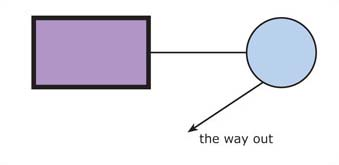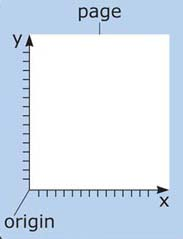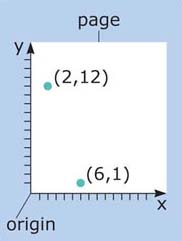Digital & Computing

### Become an OU studentCrossing the boundary: analogue universe, digital worlds

Start this free course now. Just create an account and sign in. Enrol and complete the course for a free statement of participation or digital badge if available.

# 4.8 Interlude – diagrams

Some types of visual information can be represented more economically than in a bitmap. Consider the rather pointless little diagram shown in Figure 21.

## SAQ 15Figure 21 A diagram of simple lines and shapes

Why do you think storing such a diagram as a bitmap would be a waste of memory?

The huge majority of the pixels will just be white, the background to the picture. The only information all these white pixels give us is the simple fact that the background colour is white.

So what information do we need to record about the diagram? In fact, what we really care about are the objects depicted – the rest of it is just uninteresting empty space. Our example contains five types of object: a circle, a rectangle, a line, an arrow and a piece of text. To reconstruct the diagram the only information we really need about each of these objects is:

• what sort of object it is (e.g. line, square);

• its size and position on the page;

• details about its colouring, width of line (line weight), and so on.

Remembering that our aim is to express Figure 21 as a set of numbers, we can now go into a bit more detail.

First of all, I'll assign a number to each type of object. Quite randomly, I'm going to choose 17 to represent a circle, 24 to stand for a rectangle, 26 for a line, 27 for an arrow and 11 for a text area. So the initial set of numbers will be:

17, 24, 26, 27, 11.

The order is not important.

Now let's record the size and position of each object. Here we need some way of identifying positions on the page. To do this we use the very simple, although slightly intimidating sounding, technique of Cartesian coordinates. (Named after Rene Descartes, who invented the system in the seventeenth century). We simply place two axes, x and y at right angles on the page, as in Figure 22.Figure 22 Cartesian coordinates, x- and y-axes

Now we can identify any point on the page by its x- and y-coordinates, simply by measuring how far from the origin along the x-axis it is and then how far along the y-axis. So, any point on the page is located by two numbers – conventionally, × always goes first – as illustrated in Figure 23.Figure 23 Two points identified by their coordinates

Now I can identify the size and position of each object in Figure 21. Exactly how this is done depends on the object, as follows.

• Starting with the circle, all I need is its radius and the position of its centre – this tells me everything I need to know about the size and location of a circle. Let's say the radius of the circle in Figure 21 is 2 cm and the position of the centre (13, 6).

• For the rectangle, I will have to record two sets of values: the position of the top left-hand corner and the position of the bottom right-hand corner. Let's say they are (1, 8) and (6, 4), respectively.

• For the line and the arrow, I simply need to record their start and end positions. I estimate the line in Figure 21 starts at roughly (6, 6) and ends at (11, 6). The arrow's start and end points are approximately (12, 4) and (9, 2).

• As for the text area, I just need the position of its top left-hand corner, which I put at about (10.5, 2.5)

Now I can put these together and produce the following intermediate set of numbers:

17, 2, 13, 6 (circle)

24, 1, 8, 6, 4 (rectangle)

26, 6, 6, 11, 6 (line)

27, 12, 4, 9, 2 (arrow)

28, 11, 10.5, 2.5 (text area)

I have not quite finished. For the whole diagram we need to identify the background colour, which is white in this case. Coding white as 255, 255, 255 in the RGB model gives us binary 111111111111111111111111, which is decimal 16,777,215.

Now, for each object, I can record such details as the line colour, fill colour, line width. I want to keep it simple, so let's say:

• the circle's line width is 1 pixel, its line colour is black (decimal 0) and its fill colour is light blue (decimal 828,124);

• the rectangle's line width is 3 pixels, its line colour is black (decimal 0) and its fill colour is a restful light purple, whose RGB value is decimal 1,340,877;

• the arrow and the line, both have a line width of 1 pixel, and their line colour is black (decimal 0);

• the colour of the text in the text area is black (0 again).

We haven't yet recorded what the text says! Easy. You remember your Unicode standard perfectly, so you know the text ‘the way out’ translates to 116, 104, 101, 32, 119, 97, 121, 32, 111, 117, 116.

Now we can put all this together and produce a final set of numbers, as follows:

• 16777215 (background colour)

• 17, 2 13, 6, 1, 0, 828124 (circle)

• 24, 1, 8, 6, 4, 3, 0, 1340877 (rectangle)

• 26, 6, 6, 11, 6, 1, 0 (line)

• 27, 12, 4, 9, 2, 1, 0 (arrow)

• 11, 10.5, 2.5, 0, 116, 104, 101, 32, 119, 97, 121, 32, 111, 117, 116 (text area)

This is a simplification, but I hope you get the idea. This sort of encoding of visual information is usually known as vector graphics, as opposed to the bitmap approach we discussed earlier which is often called raster graphics.

The obvious advantage of the vector strategy is that it is a very compact form of coding. With just 41 numbers (about 984 bytes), we have summed up the essence of our diagram. A bitmap of the same diagram would have required at least tens of thousands of bytes.

Another advantage of vector graphics is the resulting image is scalable: we can easily shrink or stretch the size of it without any loss of information.

As always in computing, though, there are problems. First, vector coding only really works with fairly simple images. It would be hopeless for the Vermeer or Signac paintings, for example. So it is only a partial answer to the problem of size. Second, if I send the set of numbers we generated to a friend, she will need a sophisticated computer program on her computer to interpret the numbers and display them as a diagram. For the moment, we may just note that programs that allow us to draw and display vector graphics are generally referred to as drawing packages. Systems for constructing and displaying raster graphics are usually called painting packages. There are many examples of each on the market.